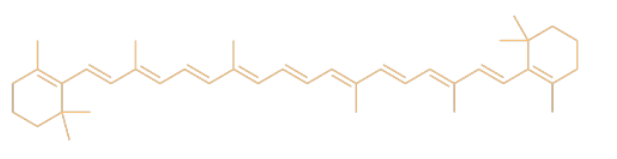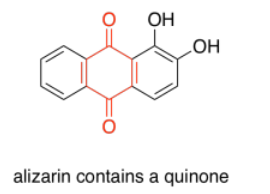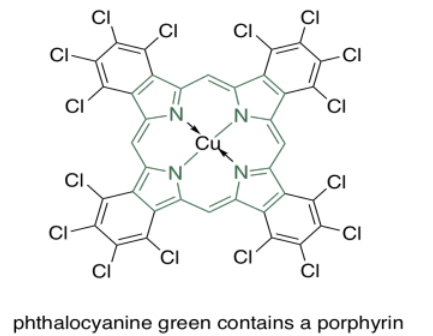Skip to main content

# 2.3: UV-Visible Spectroscopy of Organic Compounds

Many of the colors that we see in nature come not from simple atoms, but from molecules. Organic compounds -- carbon-based compounds, usually made by living things -- are sometimes very brightly colored. If you look out on an autumn day and see a woman in blue jeans walking beneath an orange maple, then you are observing a couple of organic compounds.

The blue color of her jeans is probably indigo, a dye known since antiquity, but often produced synthetically today. It still has the same color, but it is produced by factory workers rather than field hands.Figure $$\PageIndex{1}$$:

The color of the leaves comes from a mix of different compounds such as carotene. Carotene is a member of a huge class of natural products called terpenoids.Figure $$\PageIndex{2}$$:

Exercise $$\PageIndex{1}$$

What color of light is absorbed by (a) the blue jeans? (b) the leaves?

Answer a:

blue

Answer b:

orange

In most cases, the reason for the color is tied to one phenomenon in these compounds: conjugation. Conjugation is a specific pattern in the electronic arrangement of molecules. A Lewis structure of a conjugated molecule shows alternating single and double bonds between carbon atoms. In some cases, there might also be oxygen or nitrogen atoms involved in these alternating double and single bonds.

It turns out that conjugation has a very predictable effect on the energy levels of the electrons involved in those alternating bonds. It smears out the energy levels. Some of them are pushed a little higher in energy. Some drop a little lower. Many of them become spread out in between. As a result, the gaps between those energy levels becomes smaller and smaller as the amount of conjugation increases in a molecule.Figure $$\PageIndex{3}$$:

So, if we start to measure the UV spectra of a bunch of compounds, we start to see evidence of that conjugation phenomenon from the indigo and carotene. Each time we add a double bond to a conjugated system, the wavelength of light absorbed increases. It's a significant increase: maybe forty or fifty nanometers for the first double bond or two that we add in conjugation, although the differences would get a little smaller if we kept adding more of them.

 λmax (nm) C=C 170 C=C-C=C 220 C=C-C=C-C=C 260

Exercise $$\PageIndex{2}$$

Benzoic acid has an absorption maximum at 230 nm. Where do you expect to see the absorption maximum in cinnamic acid?Figure $$\PageIndex{4}$$: Answer Add another 30 or 40 nm and you get to 270 or 280 nm.

Exercise $$\PageIndex{3}$$

Polyaromatic systems have rather complicated UV spectra, but as each additional aromatic ring is added, a shift of about 30 nm occurs in the absorption maximum of the most prominent peak. If naphthalene has λmax at 220 nm, where would you see λmax for anthracene and tetracene?Figure $$\PageIndex{5}$$: Answer a: about 250 nm Answer b: about 280 nm

Conjugation is responsible for much of the visible absorption by organic compounds because the energetic spacing between π and π* orbitals falls within the same energy range as visible light. As a result, electrons can be excited from a π to a π* level when that visible light is absorbed.

There are other electrons (other than the ones in the π bonds) that can be excited by absorption of visible light. If the molecule has sigma bonds, there is always a possibility of a σ to σ* transition, in which an electron in a σ bond gets excited to the antibonding level. If the molecule has lone pairs, there could be n to σ* transitions or n to π* transitions (n is nonbonding).

However, none of these absorb light as strongly as π to π* transitions. Just as there were differences in the strength of absorbances (extinction coefficients) in transition metal compounds (where MLCT absorbed light strongly, but d-d transitions absorbed light only weakly), we see large extinction coefficients for π to π* but only tiny coefficients for σ to σ* transitions, for example. These differences are related to the spatial relationships between the two orbitals involved in each case, rather than the energy differences.Figure $$\PageIndex{6}$$:

Exercise $$\PageIndex{4}$$

Organic compounds, like transition metals, can have absorptions of varying strengths. Consider the following table for quinone:Figure $$\PageIndex{7}$$:
 λ (nm) ε (cm2 mol-1) 240 24 000 280 400 430 20
1. Use the data table for quinone to sketch a UV spectrum.
2. What color is quinone?
Answer a:Answer b:

yellow

Structures that are very good at absorbing visible light are sometimes called chromophores. There are plenty of common chromophores in nature, such as that extended terpenoid structure seen in carotenes; a similar structure is found in lycopene, which gives tomatoes their red color.

Quinones are also very commonly seen in chromophores. In humans, the most common chromophore is melanin; its brown color is derived from a quinone-based structure. A different quinone structure, alizarin, is a dye known since antiquity; it was historically derived from madder root.Figure $$\PageIndex{8}$$:

Porphyrins are also very common in humans and other organisms. They are found in compounds such as hemoglobin, known more for their oxygen-carrying ability and other indispensible properties than their color. However, they are quite brightly colored. Interestingly, the colors of porphyrins and other chromophores can be tuned quite easily through variation of side groups around the main structure. Phthalocyanin green, for example, has the same porphyrin structure as blood-red heme, but is modified by additional aromatic rings and chlorine atoms, and contains a copper rather than an iron. These color-modifying groups are called autochromes.Figure $$\PageIndex{9}$$:

The anthocyanins are another widespread group of naturally-occurring compounds. Like porphyrins and quinones, they contain a variety of autochromes; the groups arranged around the central, three-ring structure that forms the essential chromophore of the anthocyanins. Variations among these side groups leads to a variety of colors ranging from red to blue. The one shown below gives a purple color to the flowers -- petunias -- in which it is frequently found.Figure $$\PageIndex{10}$$:

One last group of common chromophores is not found in nature at all. These are the azo dyes, discovered in the 19th century and still in widespread use today.Figure $$\PageIndex{11}$$:

Note that the effects of autochromes can be somewhat complicated in tuning the absorption of the main chromophore. Not only does the identity of the side group have an influence (such as the sulfonate group, SO3-, or an amine group, NH2, in an azo dye), but the group's exact position is important as well. There is actually a list of rules (Woodward Rules) that can be used to predict absorption maxima of conjugated systems, but we won't go into those here.

Exercise $$\PageIndex{5}$$

In each of the following pigments,

1. circle the main chromophore.
2. identify the class of chromophore.
3. predict the observed color.Figure $$\PageIndex{12}$$:Figure $$\PageIndex{13}$$: Answer